January 1, 0001

# Random Walk

## Introduction

This lab features a random walk simulation that answers the following question: Given the radius of a circular room, how many random steps does it take to reach to reach the wall from the center? To explore this, we will look at the distribution of steps required and describe it in terms of a discrete probability distribution. Later on, we will look at the radius parameter and find relationships between it and the different summary statistics that we compute (mean, median, range, variance, skewness).

The paper will start off with the methods used in this lab. The programming language R was used and a simulation was created to simulate a random walk according to the description above. It will also discuss the methods used to find the discrete probability distribution that best fits the data and how the relationship between the radii and the summary statistics were found using regression.

After discussing the methods, the paper goes into the results. The discrete probability distribution of the data is revealed and the equations of the different summary statistics based on a regression of the radius is shared.

## Methods

### Obtaining the distribution of steps required

The simulation written performs the following steps given the size of a room:

1. Start off in the center of the room
2. Calculate a random angle $\theta$
3. Step in the X direction by $cos(\theta)$
4. Step in the Y direction by $sin(\theta)$
5. Repeat steps 2-4 until the wall is reached

The number of steps required is then recorded into a vector and the simulation is performed 999 more times. This gives us the distribution of steps required to reach the wall given the room size.

### Computing summary statistics

Given the distribution vector pertaining to the room size, we then find the following information:

• Measures of Central Tendency: Mean & Median
• Measures of Variation: Variance & Range
• Skewness & Shape of Histogram

For every room size selected, a total of three trials of 1000 simulations each is conducted.

### Fitting the data to a distribution

Since the simulation counts the steps required before reaching the walls of the room, it describes the process of a geometric distribution. To confirm the suspicion, the function fitdistr inside the package MASS was used to find the parameter (probability) of the geometric distribution to best fit the simulation data. In this case the simulation data used is of room size 100.

The probability density histogram of the data is then shown with the overlay of the geometric distribution that best fit the data.

Another more robust way to visually see the goodness of fit of the distribution is to use what is called a Quantile-Quantile Plot (Q-Q Plot), Using the quantiles from the theoretical distribution, we compare it to the quantiles of the simulated data and check to see that it follows the Q-Q line closely.

### Finding relationships between the radii and the summary statistics

From the simulations performed, vectors have been saved containing the summary statistics for the different room sizes. Vectors are then created from these vectors as columns inside a data frame. Having this data frame allows us to use ggplot to graph the data using scatter plots.

ggplot was used as opposed to the conventional base package since it allows us to add layers to the graphs, which was used to show the geometric distribution on top of the density histogram in the previous section. ggplot is also aesthetically more pleasing as it decreases the margins and makes smart choices on the shadings of the axes on the graph.

After plotting the data, regressions are then taken with respect to different polynomial degrees of radii and analyzed for best fit using the adjusted r squared value as a means for comparison.

The adjusted r squared value is a good comparator since it tells us a proportion of how much the variation in the data is accounted for by the model.

## Results

The shape of the distribution matches the geometric distribution as shown in Figure IX & X. Figure IX shows how the probability density histogram nicely fits the overlay of the geometric distribution with the fitted parameters. The description of the problem closely matches the description of the geometric probability distribution and both distributions are skewed right. These observations increase our confidence that the distribution is a good fit. This can further be verified with the Q-Q Plot.

The Q-Q Plot in Figure X shows how the theoretical quantiles compare with the quantiles from the distribution. Since the observed sample quantiles start off in one side of the Q-Q line and ends up on the other side at the end, we can say that the data is not skewed with respect to the theoretical distribution. This along with the fact that the sample quantiles closely fit the Q-Q line supports the proposition that the geometric distribution is a good fit for the steps required in the random walk.

Regressions were then performed for each summary statistic and the models that had the lowest degree polynomial with respect to radii and with relatively small standard error were chosen.

For the mean, quadratic regression was performed and it produces the following model $$\mu(Steps) = -31.409 + 7.800(radii) + 0.837(radii^2)$$ With this model, the adjusted $r^2$ value is 99.938%, which tells us that 99.938% of the variation in means is accounted for by the quadratic regression above. It’s fit to the observed values can be seen in Figure XI.

For the median, quadratic regression was performed and produces the following model $$\widetilde{Steps} = -19.525 + 5.096(radii) + 0.687(radii^2)$$ The model above has an adjusted $r^2$ value of 99.957% which tells us that 99.957% of the variation in medians is accounted for by the regression above. It’s fit to the observed values can be seen in Figure XIII.

For the range, the quadratic regression performed creates the following model $$Range(Steps) = 63.873 - 9.073(radii) + 5.572(radii^2)$$ The model has an adjusted $r^2$ value of 99.278% which tells us that 99.278% of the variation in the ranges of steps is accounted for by the regression above. It’s fit to the observed values can be seen in Figure XV.

For the variance, the cubic regression performed creates the following model $$\sigma^2(Steps) = -88471.866 + 32256.076(radii) - 2418.835(radii^2) + 61.650(radii^3)$$ The model has an adjusted $r^2$ value of 99.779% which tells us that 99.779% of the variation in the variations of steps is accounted for by the regression above. It’s fit to the observed values can be seen in Figure XVII

Looking at the scatter plot of radii vs skewness of steps shown in Figure XVIII, there appears to be no relationship between the radii and skewness of steps. What the scatter plot suggests that the skewness is uniformly distributed.

## Conclusions

In summation, the distribution of steps required to reach the end of the wall follow a geometric distribution. This was backed up using the probability density histogram and the Q-Q Plot.

Most of the summary statistics follow a quadratic regression while the variation follows a cubic regression. Skewness are uniformly distributed across the different simulations.

In other words, the mean, median, and range follow a function that is a second degree polynomial based on the size of the room ($r^2$) and the variation follows a function that is a third degree polynomial based on the size of the room $(r^3)$

## Appendix

### Tables

#### Table I, Summary Statistics for Room Radius of 2

Trial Mean Median Variance Range Skewness
1 5.827 5.000 13.471 36.000 2.446
2 5.761 5.000 10.635 22.000 1.780
3 5.805 5.000 11.430 21.000 1.882

#### Table II, Summary Statistics for Room Radius of 3

Trial Mean Median Variance Range Skewness
1 11.566 9.000 59.055 60.000 1.854
2 11.940 10.000 74.487 68.000 2.467
3 11.242 9.000 53.417 48.000 1.922

#### Table III, Summary Statistics for Room Radius of 4

Trial Mean Median Variance Range Skewness
1 19.306 15.000 171.688 90.000 1.721
2 18.127 15.000 143.284 74.000 1.712
3 18.993 15.000 180.892 111.000 2.089

#### Table IV, Summary Statistics for Room Radius of 5

Trial Mean Median Variance Range Skewness
1 29.272 23.000 405.817 150.000 1.737
2 28.382 22.000 402.801 146.000 1.862
3 27.891 22.000 390.221 211.000 2.308

#### Table V, Summary Statistics for Room Radius of 10

Trial Mean Median Variance Range Skewness
1 110.234 87.000 6070.976 547.000 1.780
2 109.594 88.000 5928.037 542.000 1.864
3 108.398 86.000 6469.467 621.000 2.191

#### Table VI, Summary Statistics for Room Radius of 25

Trial Mean Median Variance Range Skewness
1 623.260 492.000 197161.8 3262 1.965
2 637.890 511.000 212826.6 3394 2.009
3 653.661 528.5 211126.2 3694 1.841

#### Table VII, Summary Statistics for Room Radius of 50

Trial Mean Median Variance Range Skewness
1 2507.935 1939.0 3231980 11471 1.726
2 24675.615 1992.0 3248443 14150 2.060
3 2488.795 2001.5 3038915 14689 1.843

#### Table VIII, Summary Statistics for Room Radius of 100

Trial Mean Median Variance Range Skewness
1 8983.406 7257.0 38271895 52549 2.027
2 9372.917 7537.0 41658620 51810 1.867
3 8974.549 7294.5 41868765 60326 2.420

### Figures

#### Figure I, Histogram of Room Radius 2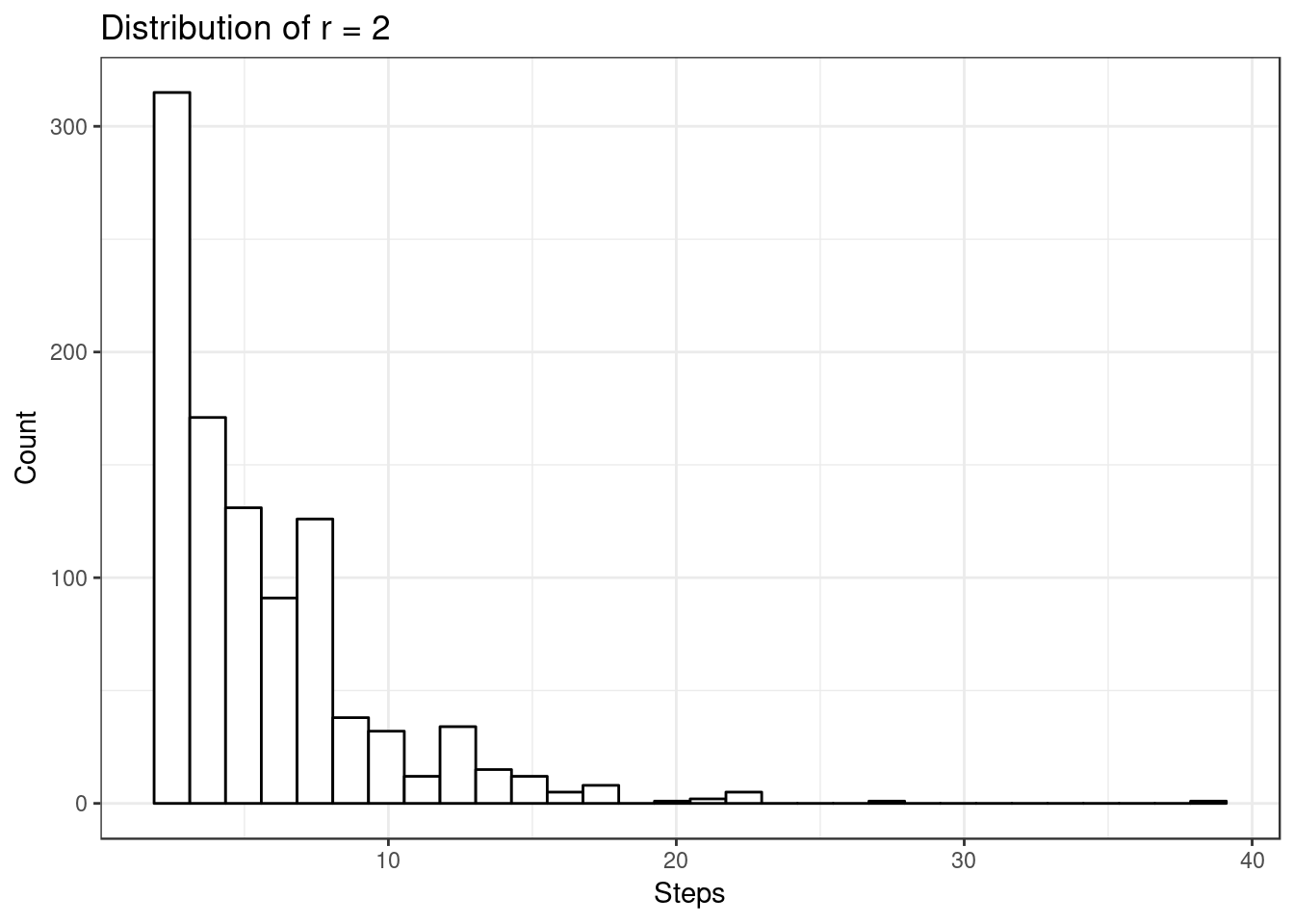#### Figure II, Histogram of Room Radius 3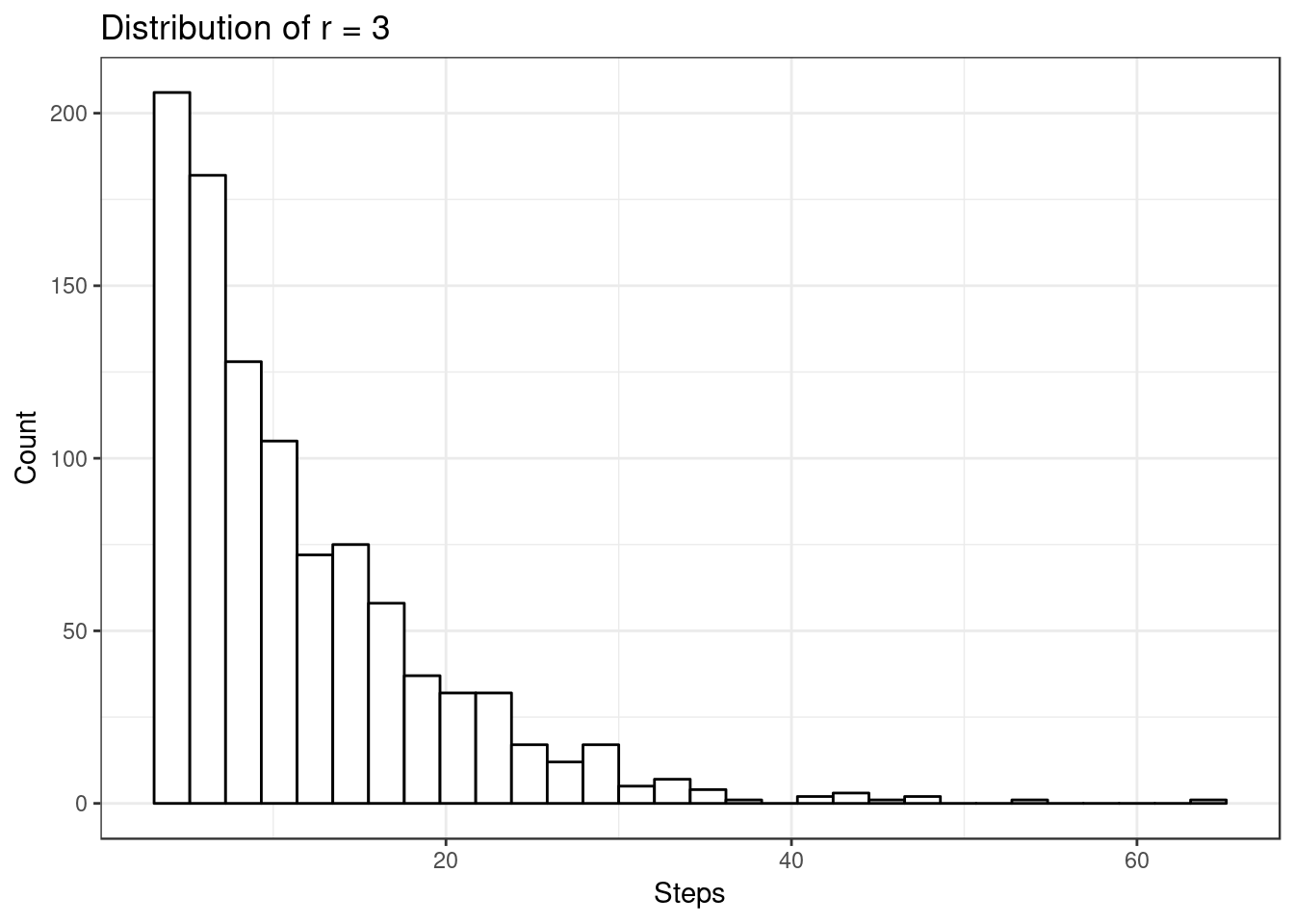#### Figure III, Histogram of Radius 4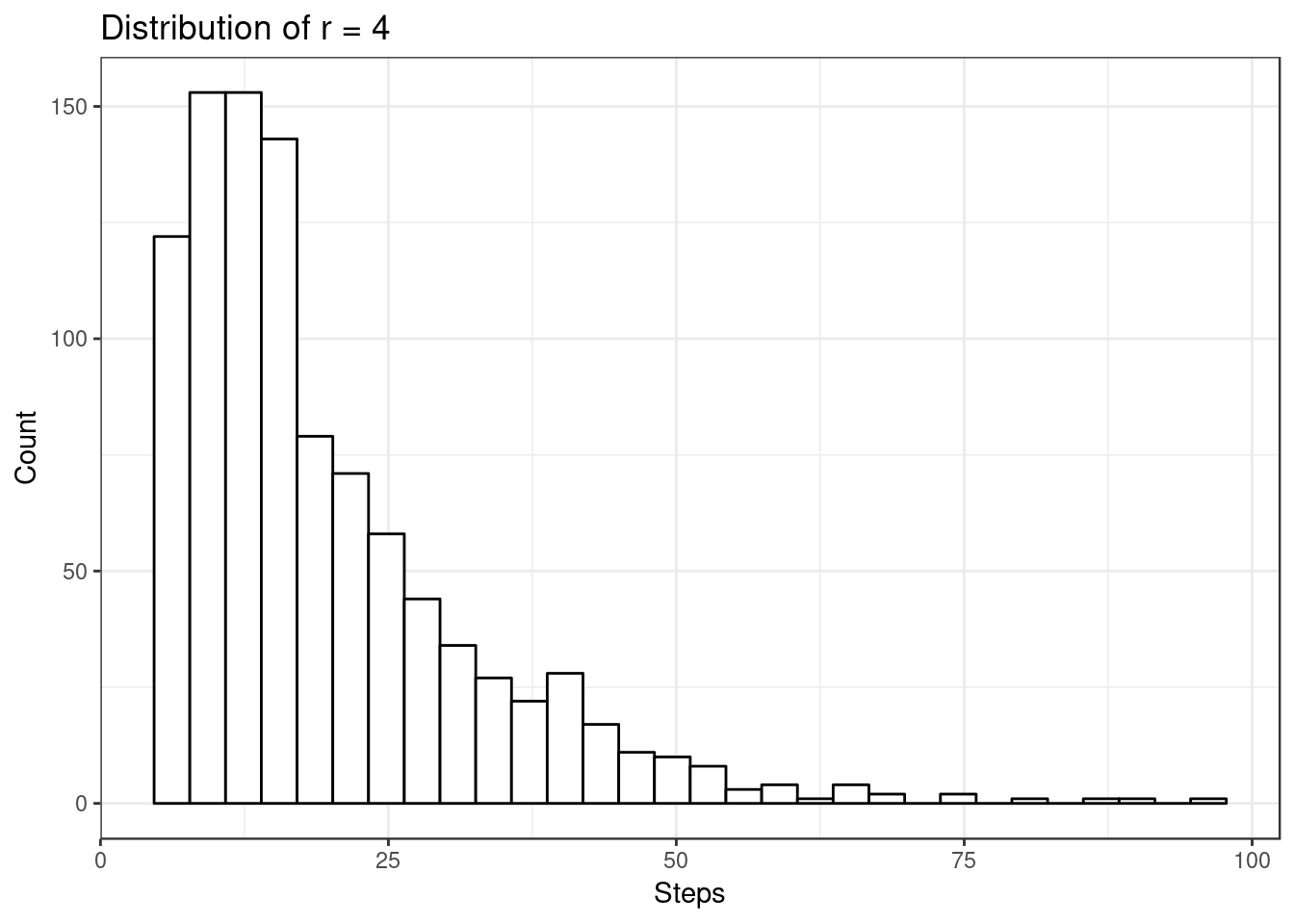#### Figure IV, Histogram of Radius 5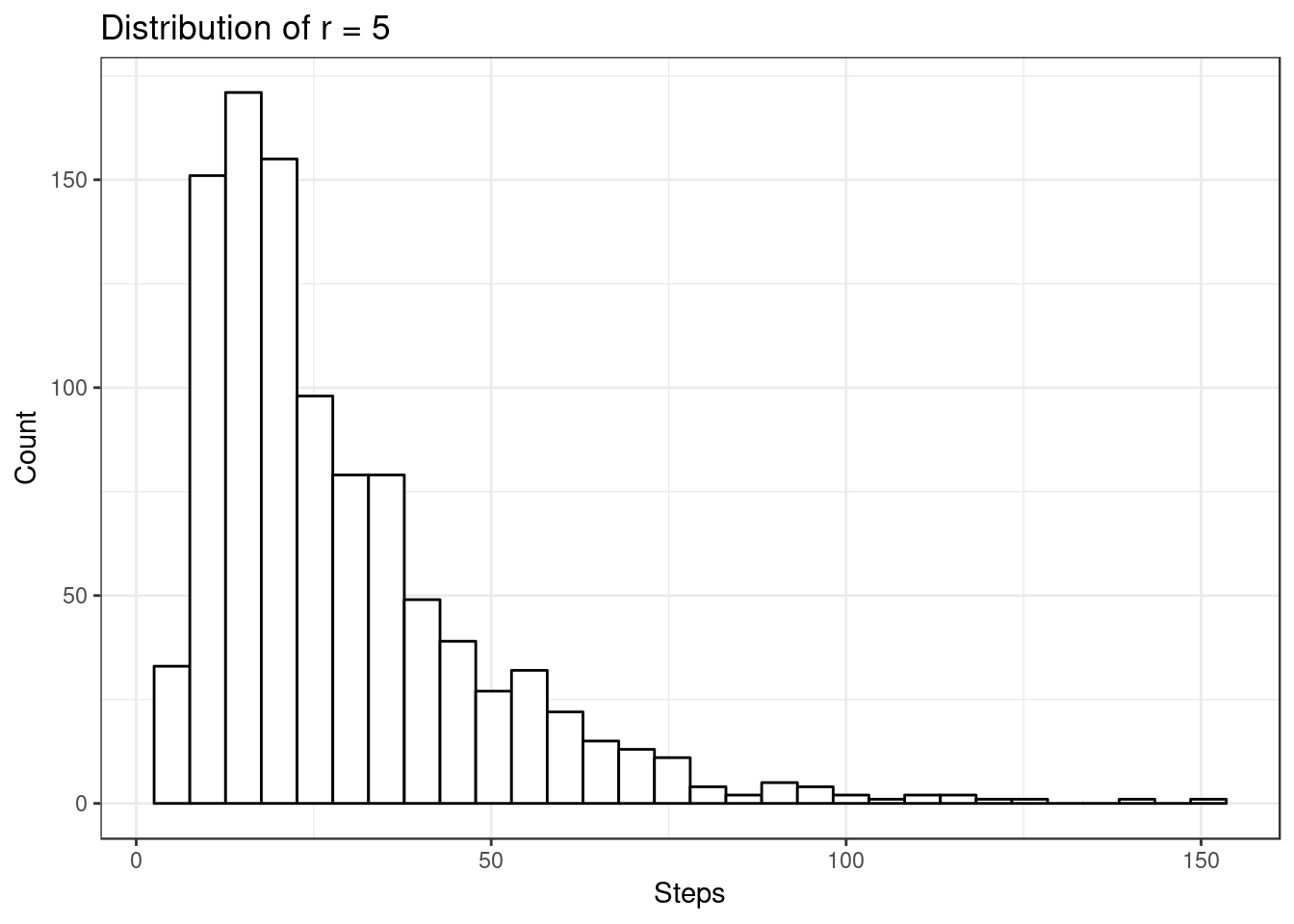#### Figure V, Histogram of Radius 10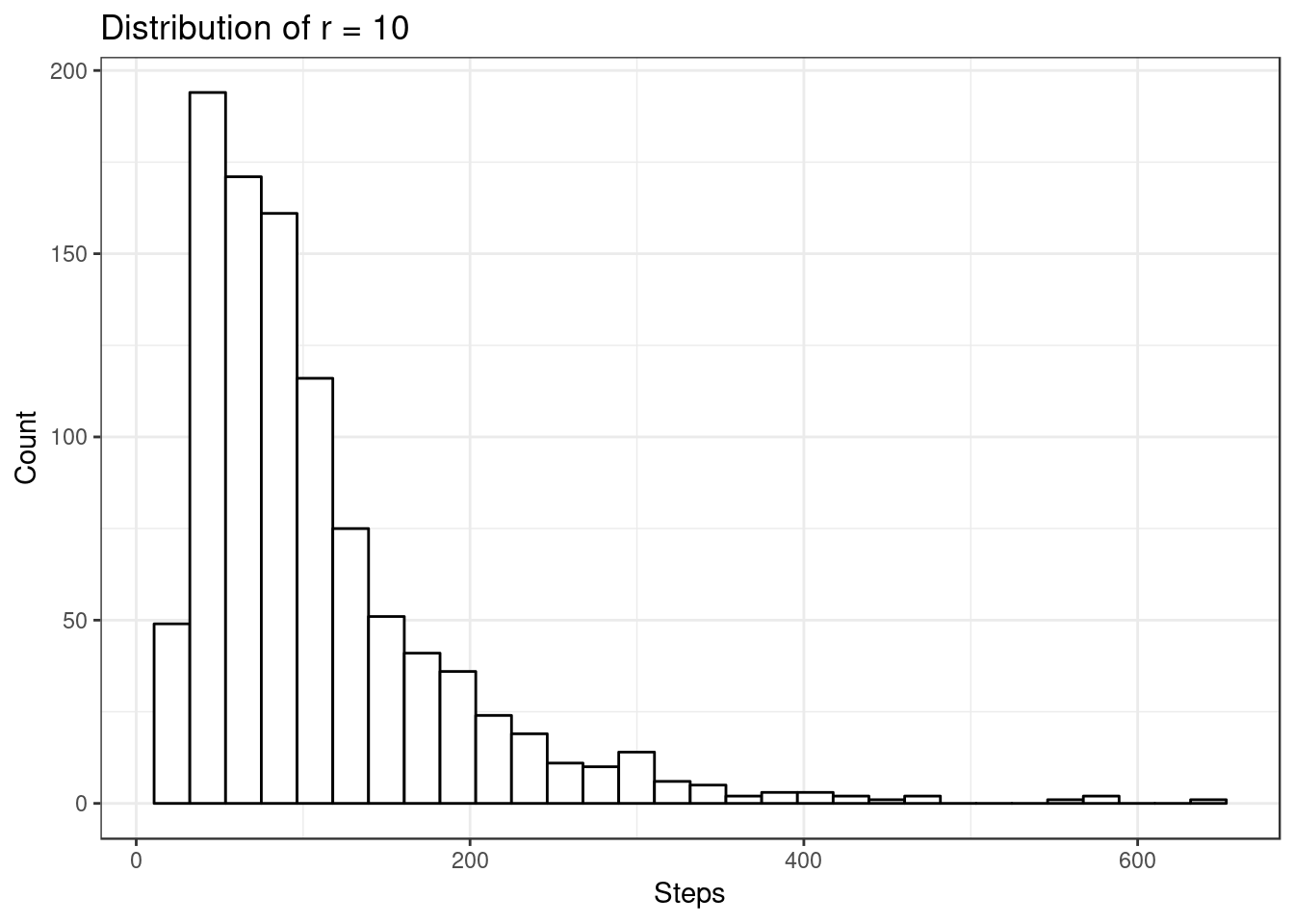#### Figure VI, Histogram of Radius 25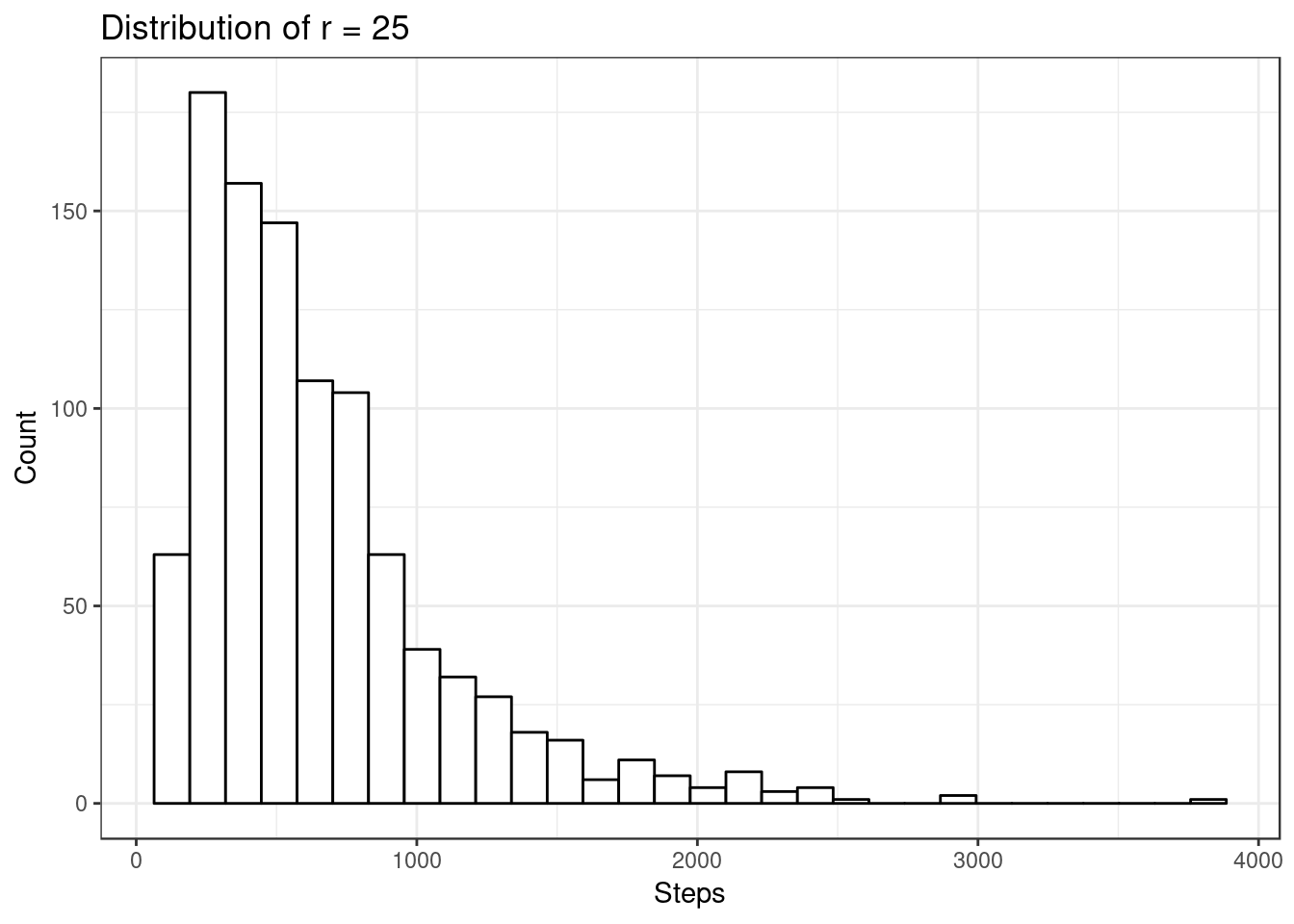#### Figure VII, Histogram of Radius 50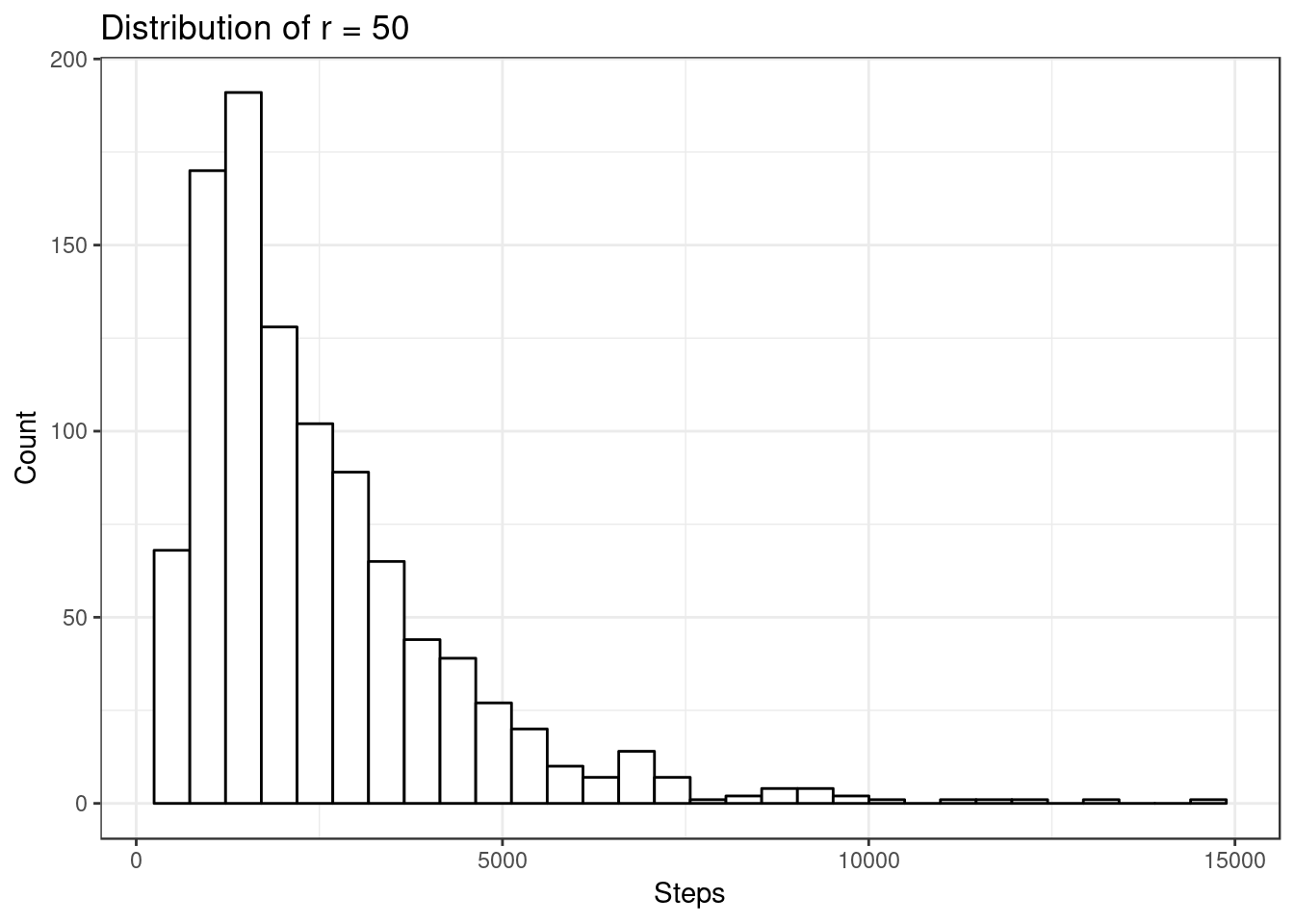#### Figure VIII, Histogram of Radius 100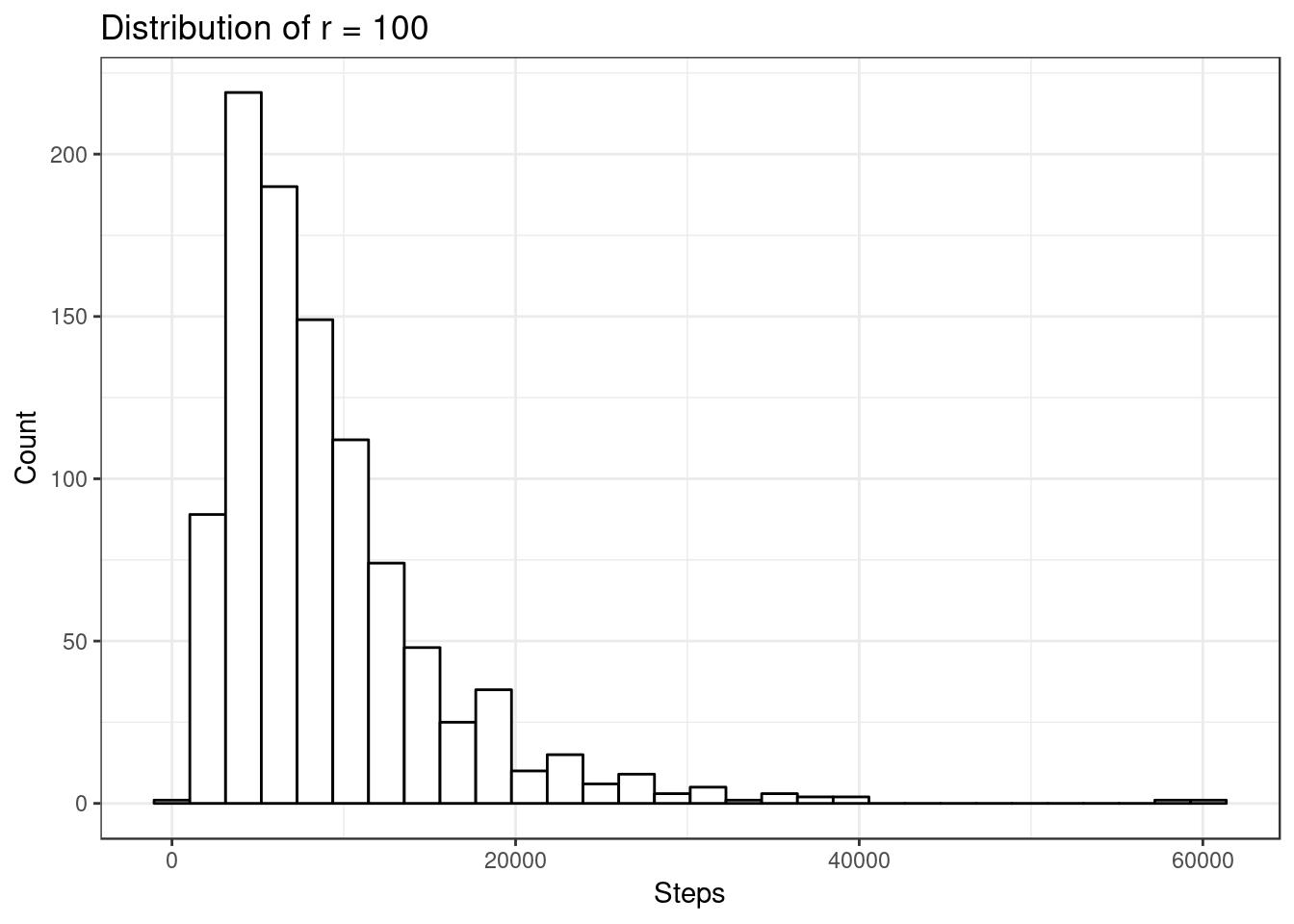#### Figure IX, Probability Density Histogram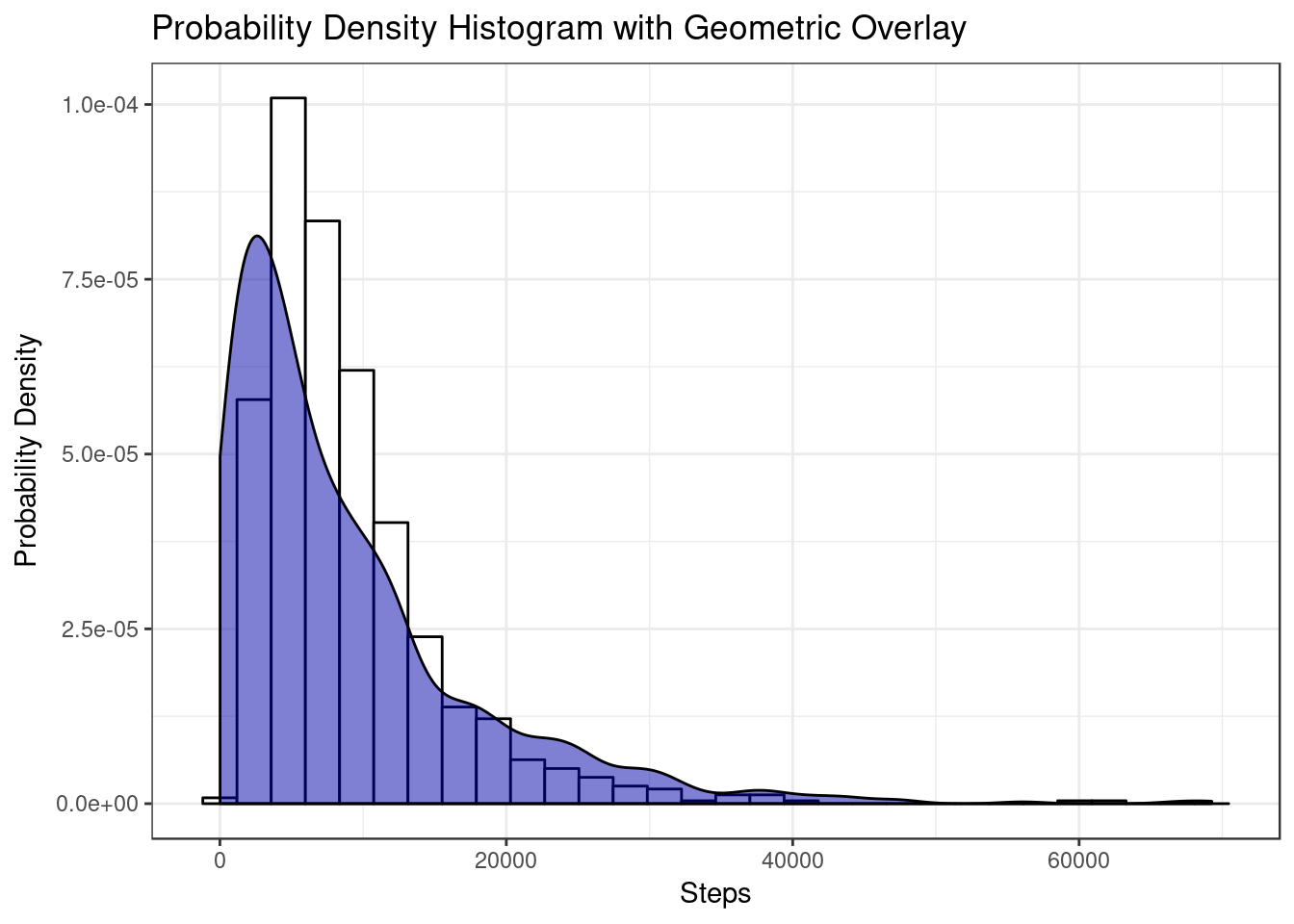#### Figure X, QQ-Plot of Theoretical vs Sample Data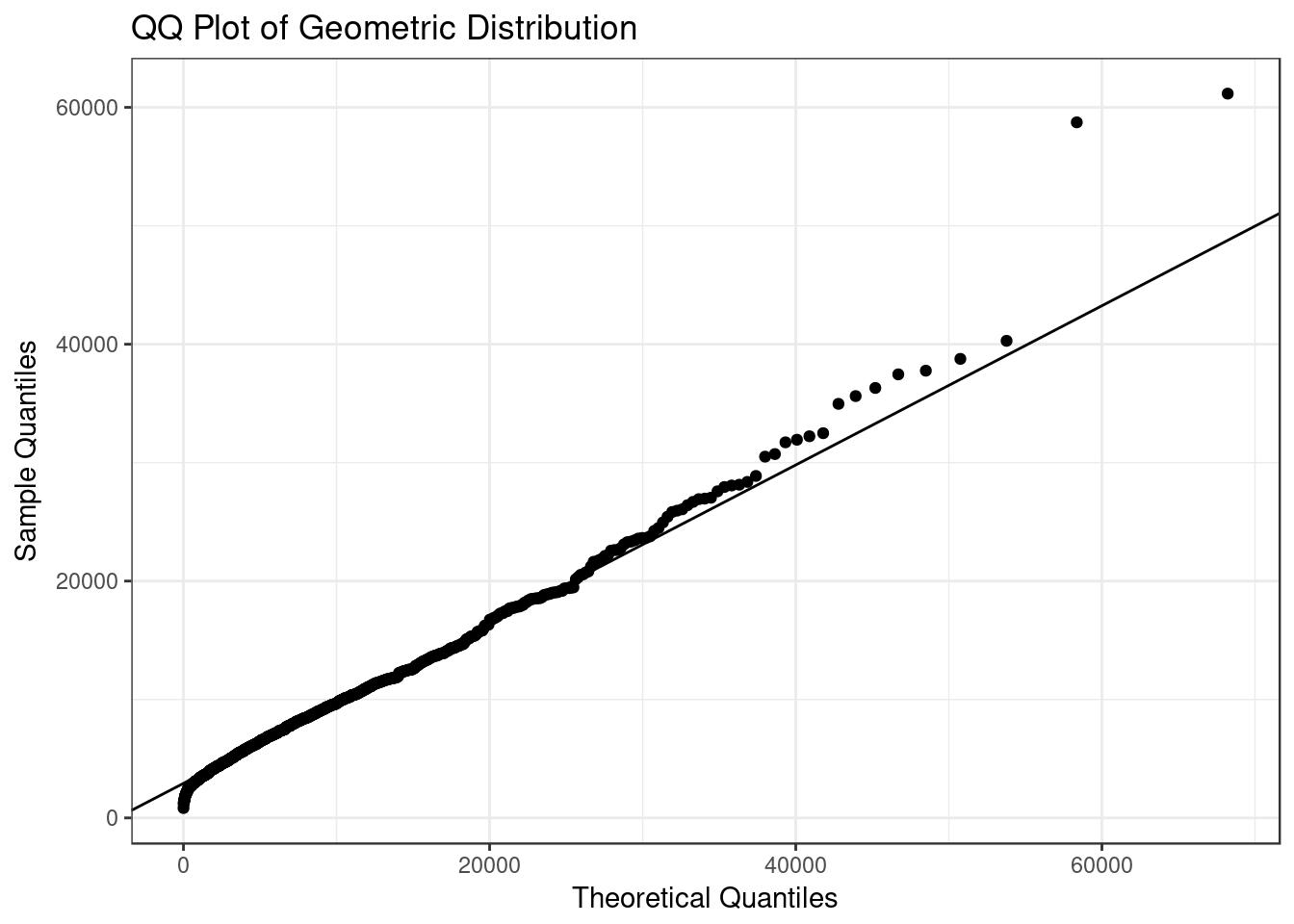#### Figure XI, Scatterplot of Radii vs Mean Steps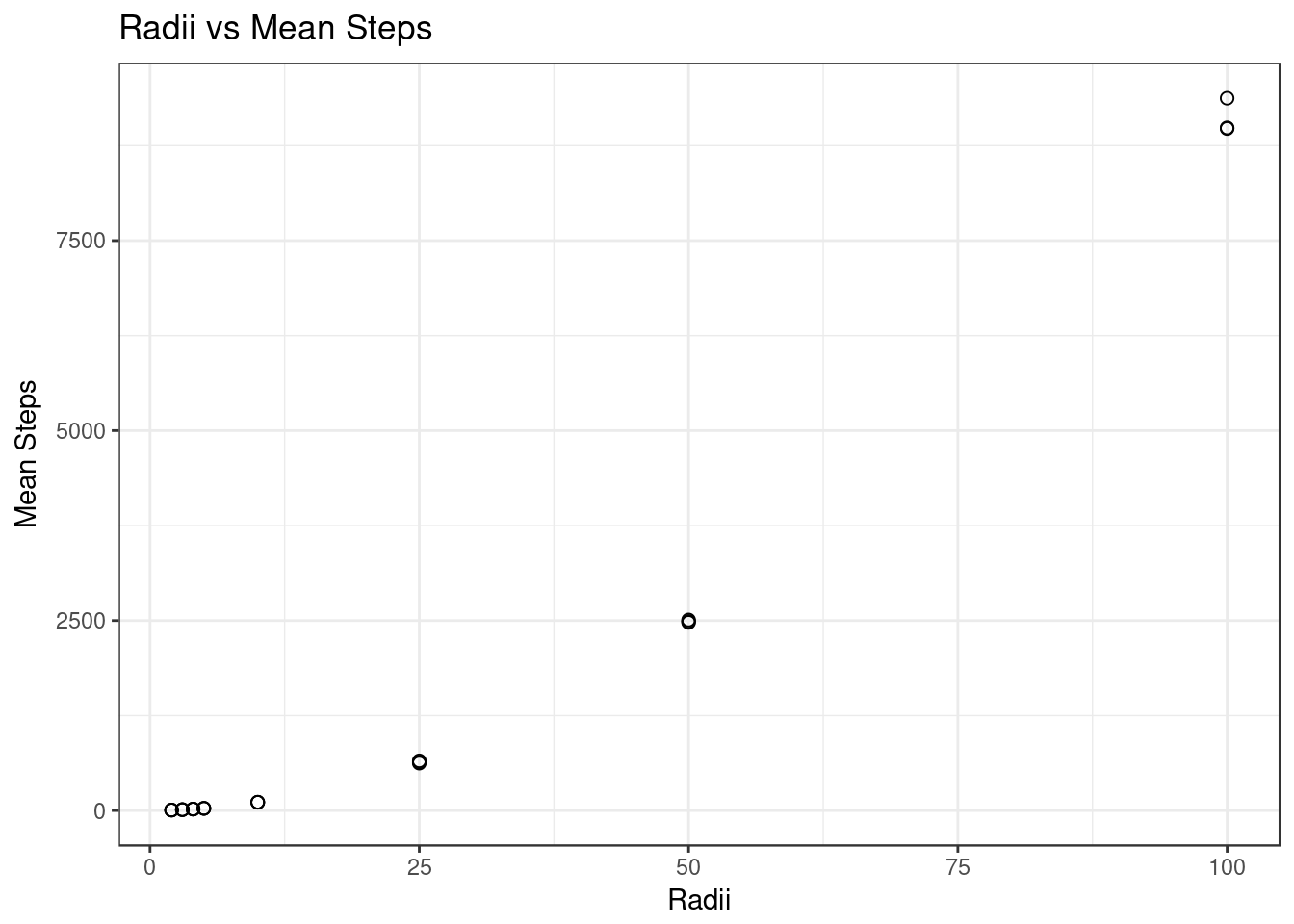#### Figure XII, Scatterplot with Quadratic Regression of Radii vs Mean Steps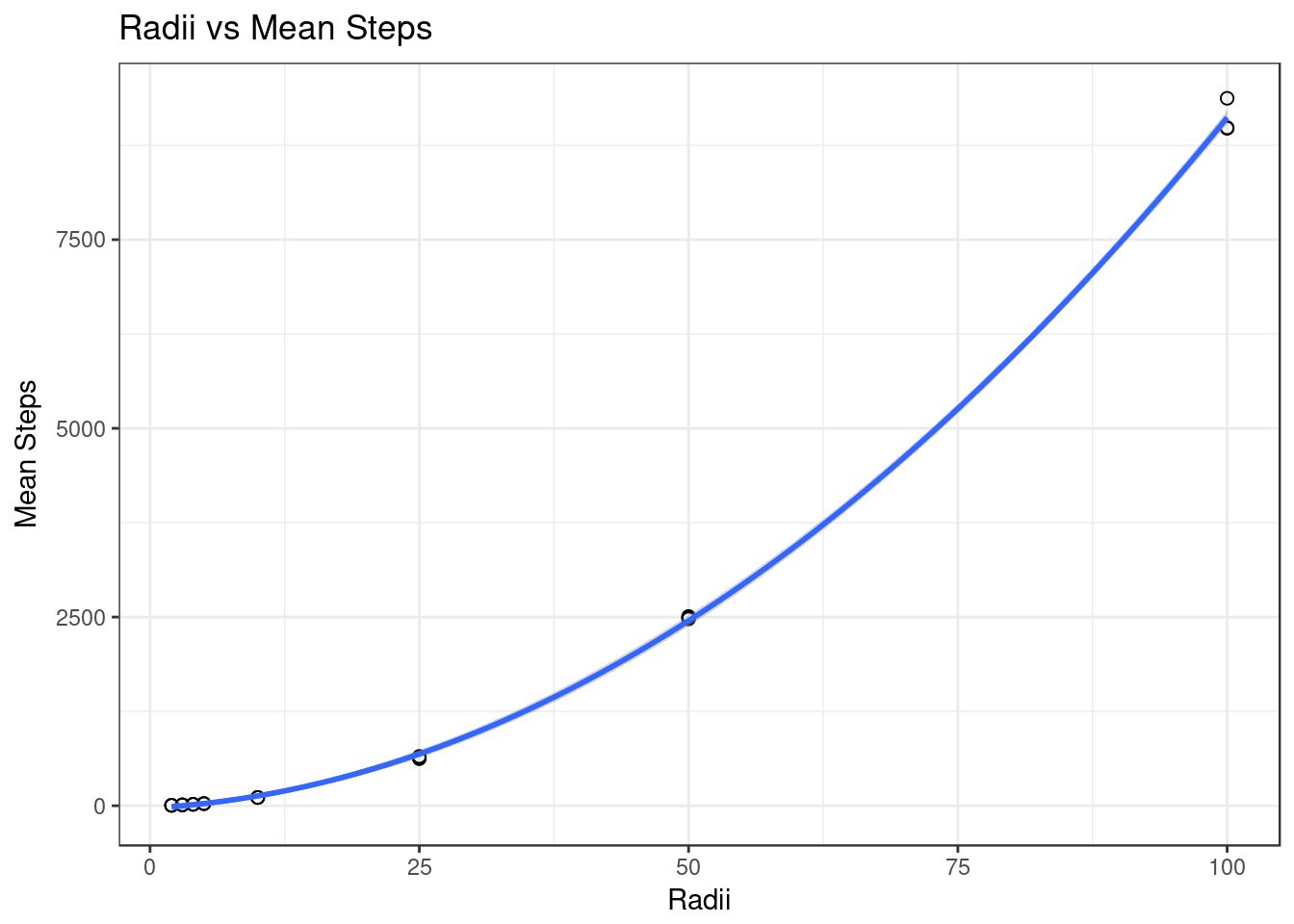#### Figure XIII, Scatterplot of Radii vs Median Steps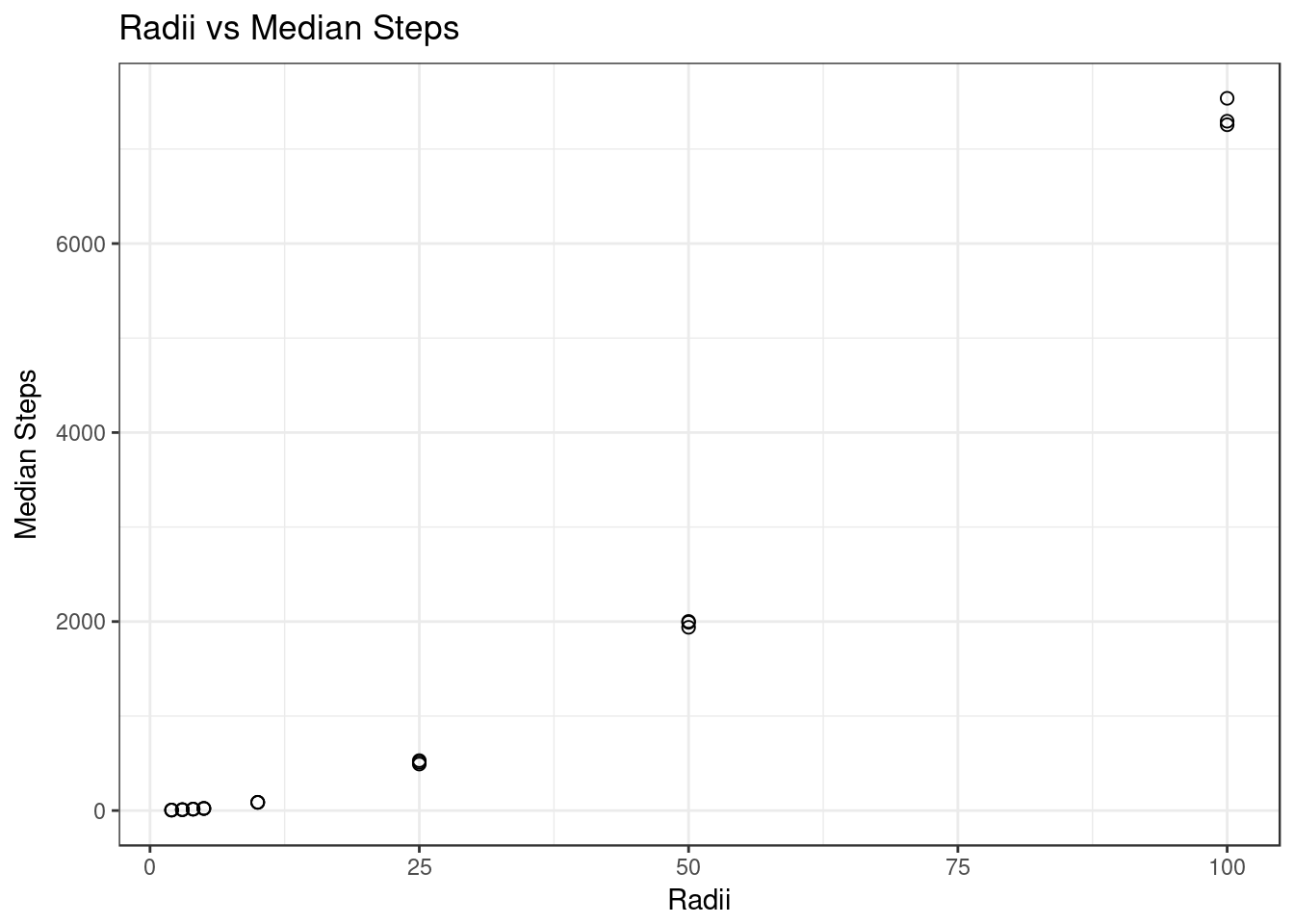#### Figure XIV, Scatterplot with Quadratic Regression of Radii vs Median Steps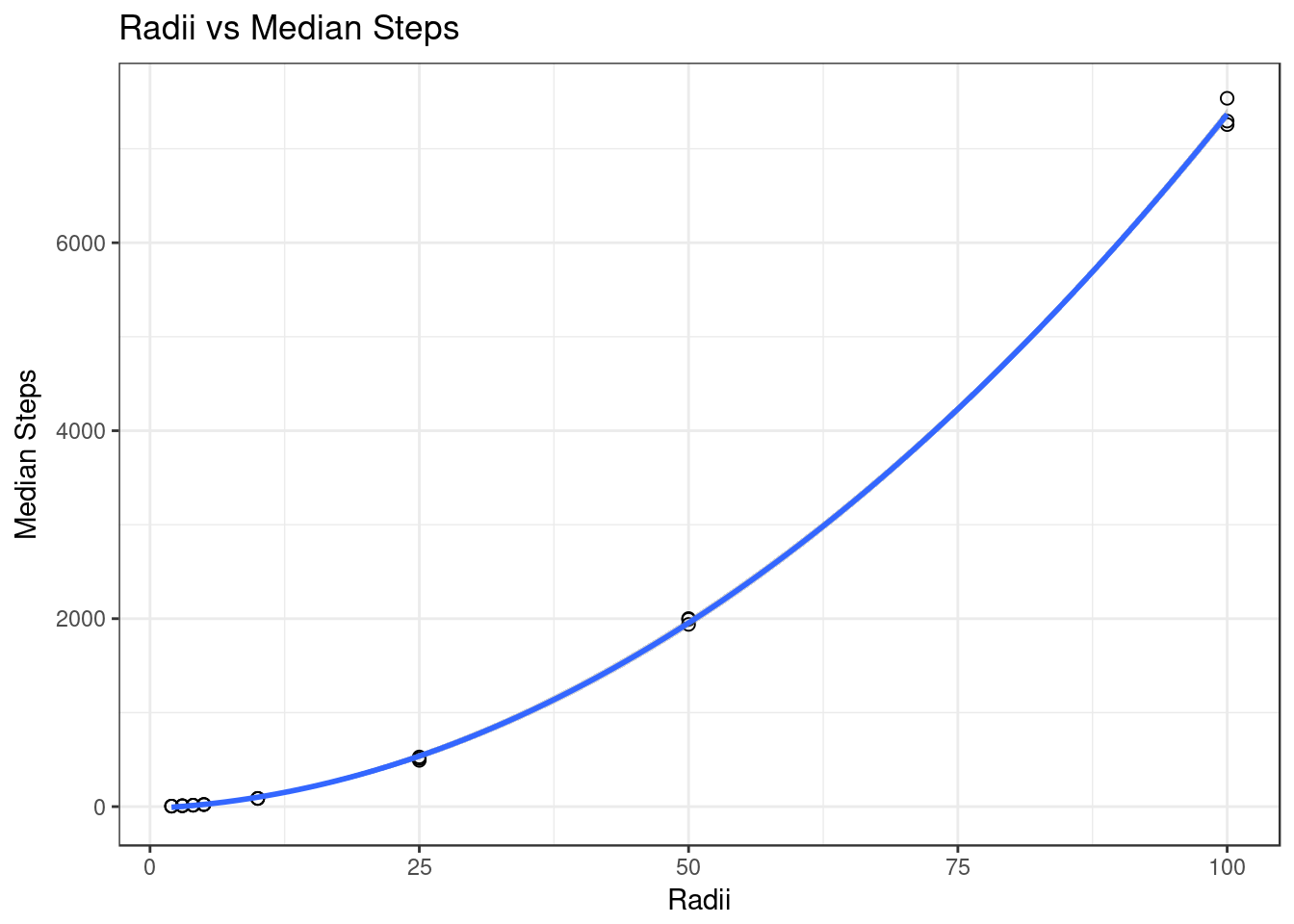#### Figure XV, Scatterplot of Radii vs Range of Steps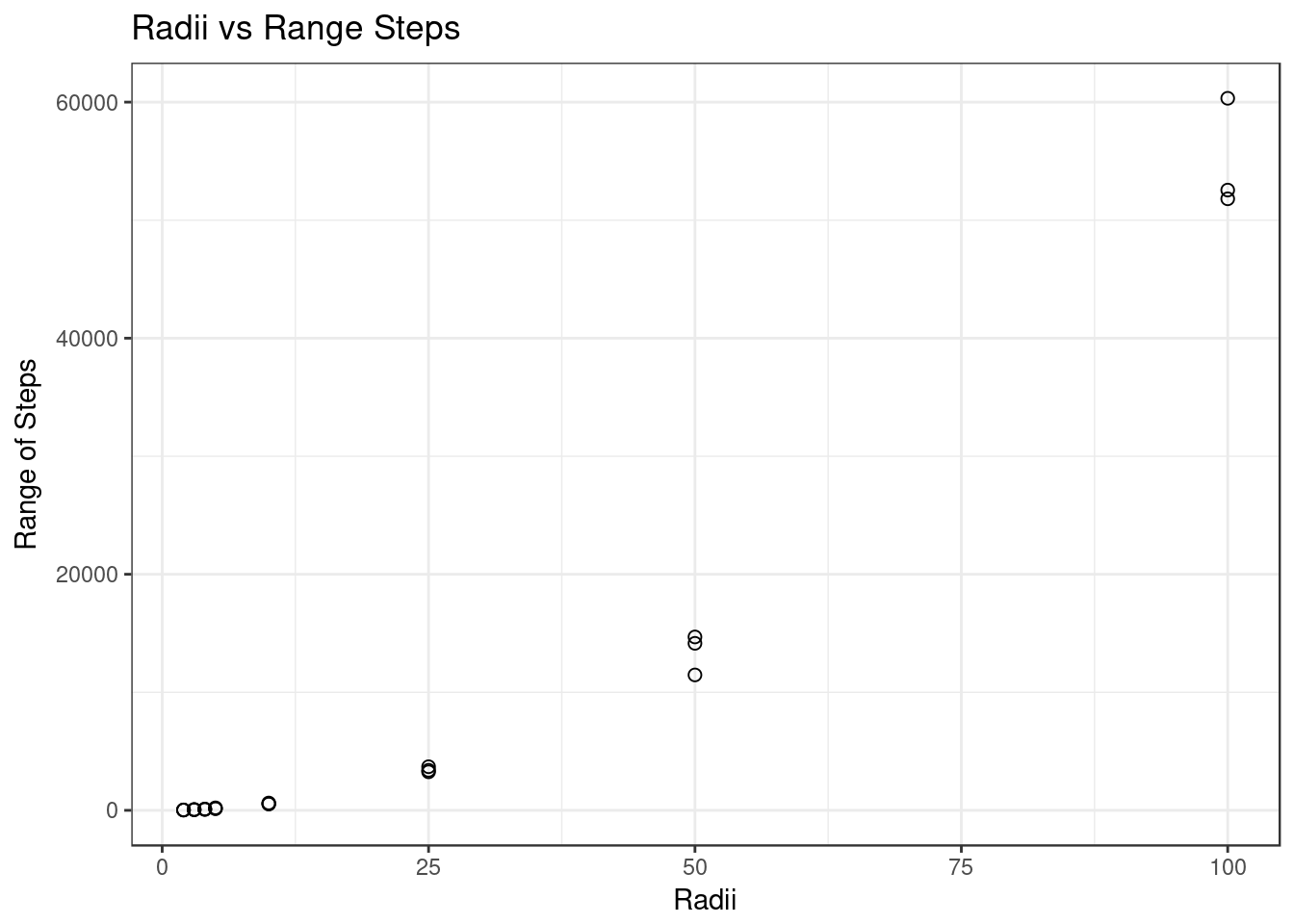#### Figure XVI, Scatterplot with Quadratic Regression of Radii vs Range of Steps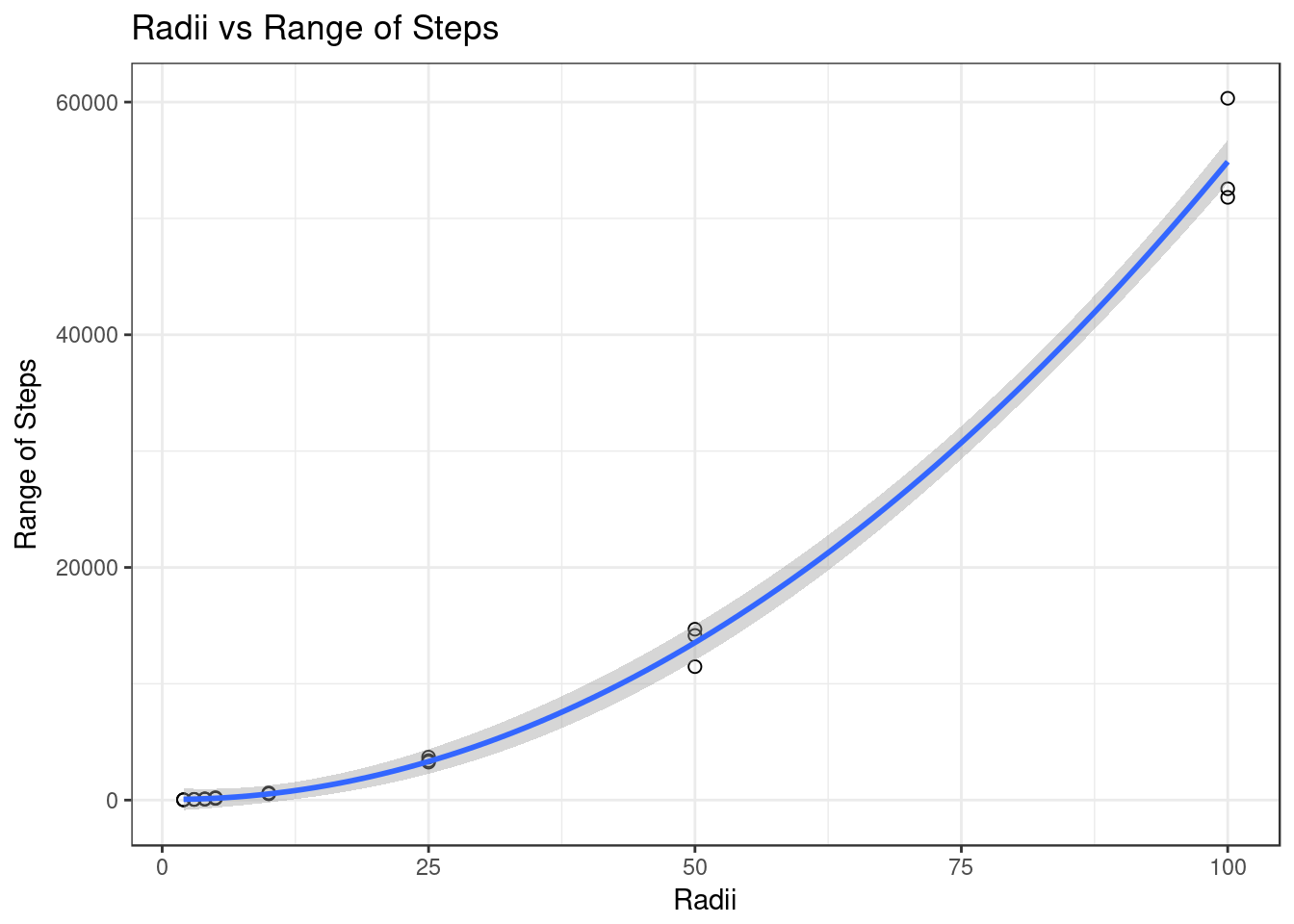#### Figure XVII, Scatterplot of Radii vs Variance of Steps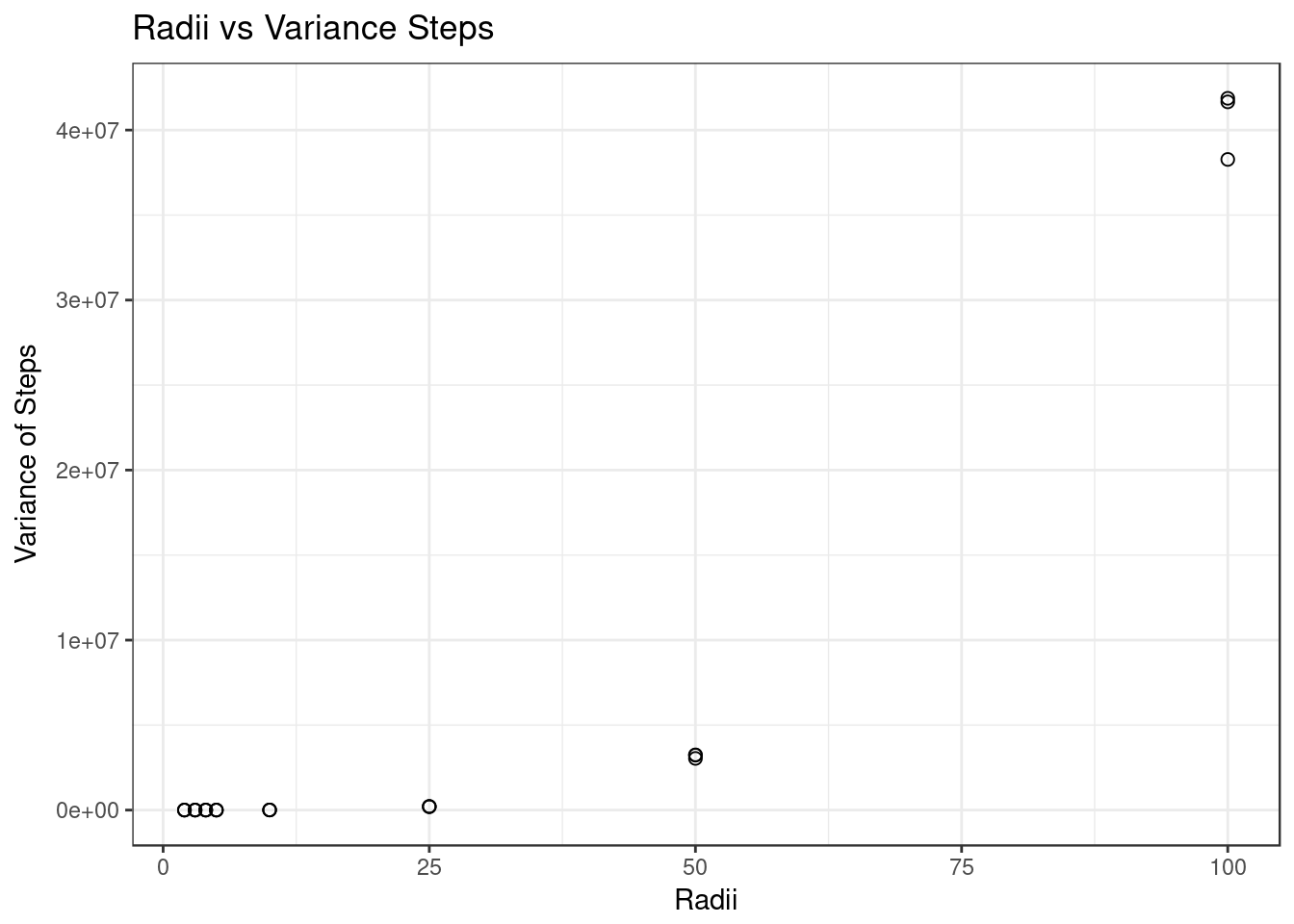#### Figure XVIII, Scatterplot with Cubic Regression of Radii vs Variance of Steps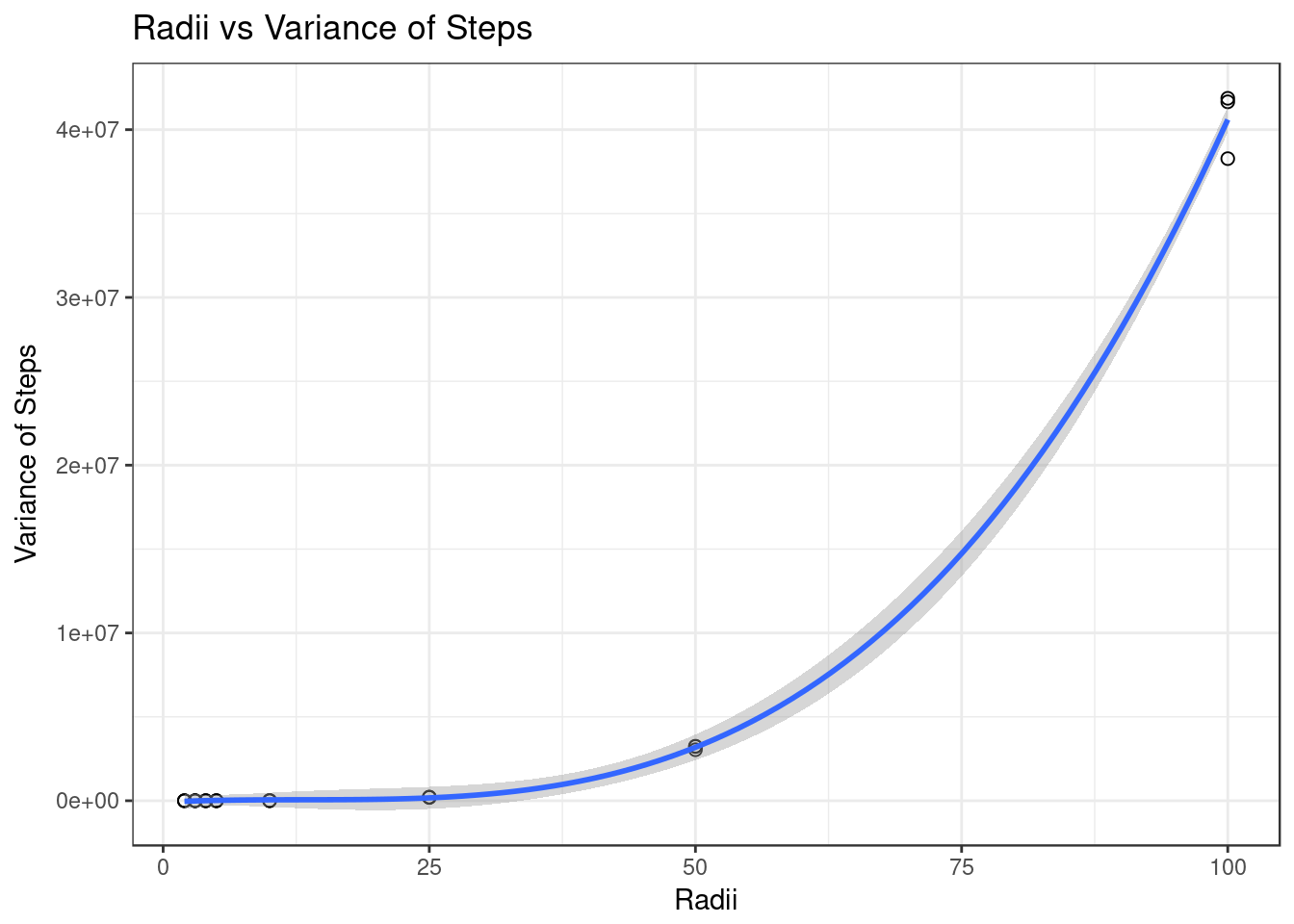#### Figure XIX, Scatterplot of Radii vs Skewness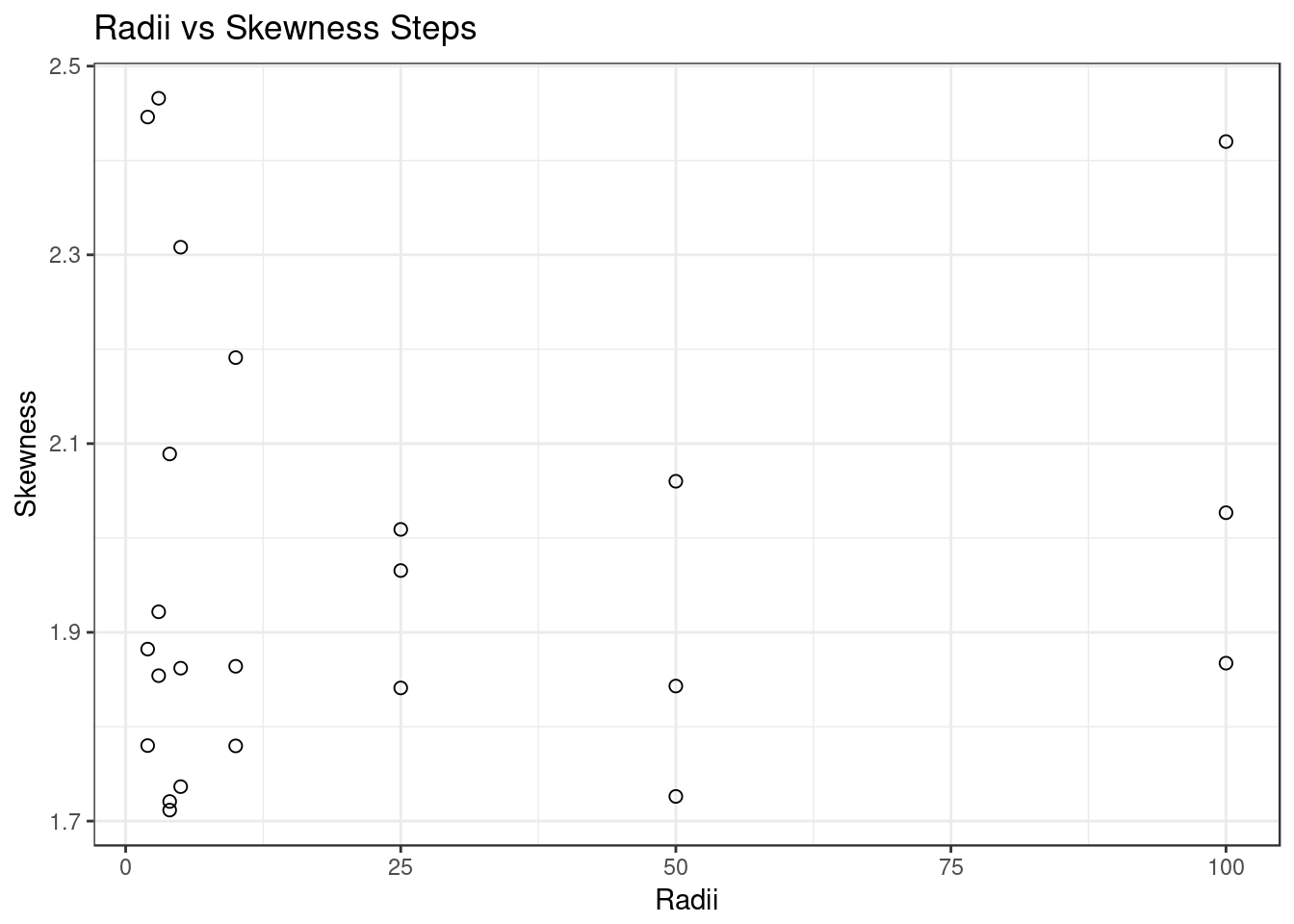### R Code

rm(list=ls())
library(moments)
library(ggplot2)

simulateRoom = function(r) {
n = 1000
results = numeric(n)
x = 0
y = 0
dist = 0
num = 0
for (i in 1:n) {
while(dist < r) {
deg = runif(1, min=0, max=360)
newx = cos(deg)
newy = sin(deg)
x = x + newx
y = y + newy
num = num+ 1
dist=(x^2 + y^2)^0.5
}
results[i] = num
x= 0
y = 0
dist = 0
num = 0
i = i + 1
}
return(results)
}

repetitions = 3
radii = c(2, 3, 4, 5, 10, 25, 50, 100)

median_stepcount = c()
mean_stepcount = c()
variance_stepcount = c()
range_stepcount = c()
skewness_stepcount = c()
for (r in radii) {
room_data = data.frame()
print(paste("Currently simulating r =", r))
for (i in 1:repetitions) {
simulation = simulateRoom(r)

## Summary Statistics
variance = var(simulation)
skewness_ = skewness(simulation)
range_data = range(simulation)

room_data_temp = data.frame(i, mean(simulation), median(simulation), variance, range_data - range_data, skewness_)
names(room_data_temp) = c("Trial", "Mean", "Median", "Variance", "Range", "Skewness")
room_data = rbind(room_data, room_data_temp)

median_stepcount = c(median_stepcount, median(simulation))
mean_stepcount = c(mean_stepcount, mean(simulation))
variance_stepcount = c(variance_stepcount, variance)
range_stepcount = c(range_stepcount, range_data - range_data)
skewness_stepcount = c(skewness_stepcount, skewness_)

simulation = data.frame(simulation)
print(ggplot(simulation, aes(x = simulation)) +
geom_histogram(color = 'black', fill = 'white') +
xlab("Steps") +
ylab("Count") +
ggtitle(paste("Distribution of r =", r)) +
theme_bw())
}
print(room_data)
}

## Show the probability distribution
library(MASS)
distribution = fitdistr(simulation$simulation, "geometric") probability_simulation = data.frame(simulation, rgeom(1:1000, distribution$estimate['prob']))
names(probability_simulation) = c("simulation", "geometric")
ggplot(data = probability_simulation, aes(x = simulation)) +
geom_histogram(aes(y = ..density..), color = 'black', fill = 'white') +
geom_density(aes(x = geometric), fill = '#0000AA', alpha = .5) +
xlab("Steps") +
ylab("Probability Density") +
ggtitle("Probability Density Histogram with Geometric Overlay") +
theme_bw()

## Calculate QQ Plot of Geometric Distribution and Sample
yquantiles = quantile(simulation$simulation, c(0.25, 0.75)) xquantiles = qgeom(prob = distribution$estimate['prob'], c(0.25, 0.75))
slope = diff(yquantiles) / diff(xquantiles)
intercept = yquantiles - slope * xquantiles
ggplot() + aes(sample=simulation$simulation) + stat_qq(distribution=qgeom, dparams = distribution$estimate['prob']) +
geom_abline(intercept=intercept, slope=slope) +
ggtitle("QQ Plot of Geometric Distribution") +
xlab("Theoretical Quantiles") +
ylab("Sample Quantiles") +
theme_bw()

## Create a dataframe
room_simulation_data = data.frame(as.vector(sapply(radii, function(x) { rep(x, repetitions)})), mean_stepcount, median_stepcount, range_stepcount, skewness_stepcount, variance_stepcount)
names(room_simulation_data) = c("radii", "mean_stepcount", "median_stepcount", "range_stepcount", "skewness_stepcount", "variance_stepcount")
######### ANALYSIS OF MEANS
ggplot(room_simulation_data, aes(x = radii, y = mean_stepcount)) +
geom_point(shape = 21, size = 2) +
xlab("Radii") +
ylab("Mean Steps") +
ggtitle("Radii vs Mean Steps") +
theme_bw()

## Quadratic Regression of Means
quadratic_mean_model = lm(mean_stepcount ~ poly(radii, 2, raw = T), data = room_simulation_data)
quadratic_mean_model_summary = summary(quadratic_mean_model)
ggplot(room_simulation_data, aes(x = radii, y = mean_stepcount)) +
geom_point(shape = 21, size = 2) +
geom_smooth(method = "lm", formula = y ~ poly(x, 2, raw = T), se = T) +
xlab("Radii") +
ylab("Mean Steps") +
ggtitle("Radii vs Mean Steps") +
theme_bw()

## Analysis of Quadratic Regression of Means
quadratic_mean_coefficients = as.vector(quadratic_mean_model$coefficients) print(paste("The quadratic regression equation is: Mean Steps =", quadratic_mean_coefficients, "+", quadratic_mean_coefficients, "radii", quadratic_mean_coefficients, "radii^2")) print(paste(quadratic_mean_model_summary$adj.r.squared * 100, "% of the variation of the means is accounted for by the Quadratic Model", sep = ""))

######### ANALYSIS OF MEDIANS
ggplot(room_simulation_data, aes(x = radii, y = median_stepcount)) +
geom_point(shape = 21, size = 2) +
xlab("Radii") +
ylab("Median Steps") +
ggtitle("Radii vs Median Steps") +
theme_bw()

## Quadratic Regression of Medians
quadratic_median_model = lm(median_stepcount ~ poly(radii, 2, raw = T), data = room_simulation_data)
quadratic_median_model_summary = summary(quadratic_median_model)
ggplot(room_simulation_data, aes(x = radii, y = median_stepcount)) +
geom_point(shape = 21, size = 2) +
geom_smooth(method = "lm", formula = y ~ poly(x, 2, raw = T), se = T) +
xlab("Radii") +
ylab("Median Steps") +
ggtitle("Radii vs Median Steps") +
theme_bw()

## Analysis of Quadratic Regression of Medians
quadratic_median_coefficients = as.vector(quadratic_median_model$coefficients) print(paste("The Quadratic Regression Equation is: Median Steps =", quadratic_median_coefficients, "+", quadratic_median_coefficients, "radii", quadratic_median_coefficients, "radii^2")) print(paste(quadratic_median_model_summary$adj.r.squared * 100, "% of the variation of the medians is accounted for by the Quadratic Model", sep = ""))

######### ANALYSIS OF RANGES
ggplot(room_simulation_data, aes(x = radii, y = range_stepcount)) +
geom_point(shape = 21, size = 2) +
xlab("Radii") +
ylab("Range of Steps") +
ggtitle("Radii vs Range Steps") +
theme_bw()

## Quadratic Regression of Ranges
quadratic_range_model = lm(range_stepcount ~ poly(radii, 2, raw = T), data = room_simulation_data)
quadratic_range_model_summary = summary(quadratic_range_model)
ggplot(room_simulation_data, aes(x = radii, y = range_stepcount)) +
geom_point(shape = 21, size = 2) +
geom_smooth(method = "lm", formula = y ~ poly(x, 2, raw = T), se = T) +
xlab("Radii") +
ylab("Range of Steps") +
ggtitle("Radii vs Range of Steps") +
theme_bw()

## Analysis of Quadratic Regression of Ranges
quadratic_range_coefficients = as.vector(quadratic_range_model$coefficients) print(paste("The Quadratic Regression Equation is: Range of Steps =", quadratic_range_coefficients, "+", quadratic_range_coefficients, "radii", quadratic_range_coefficients, "radii^2")) print(paste(quadratic_range_model_summary$adj.r.squared * 100, "% of the variation of the ranges is accounted for by the Quadratic Model", sep = ""))

######### ANALYSIS OF SKEWNESS
ggplot(room_simulation_data, aes(x = radii, y = skewness_stepcount)) +
geom_point(shape = 21, size = 2) +
xlab("Radii") +
ylab("Skewness") +
ggtitle("Radii vs Skewness Steps") +
theme_bw()

######### ANALYSIS OF VARIANCES
ggplot(room_simulation_data, aes(x = radii, y = variance_stepcount)) +
geom_point(shape = 21, size = 2) +
xlab("Radii") +
ylab("Variance of Steps") +
ggtitle("Radii vs Variance Steps") +
theme_bw()

## Cubic Regression of Variance
cubic_variance_model = lm(variance_stepcount ~ poly(radii, 3, raw = T), data = room_simulation_data)
cubic_variance_model_summary = summary(cubic_variance_model)
ggplot(room_simulation_data, aes(x = radii, y = variance_stepcount)) +
geom_point(shape = 21, size = 2) +
geom_smooth(method = "lm", formula = y ~ poly(x, 3, raw = T), se = T) +
xlab("Radii") +
ylab("Variance of Steps") +
ggtitle("Radii vs Variance of Steps") +
theme_bw()

## Analysis of Cubic Regression
cubic_variance_coefficients = as.vector(cubic_variance_model$coefficients) print(paste("The Cubic Regression Equation is: Variance of Steps =", cubic_variance_coefficients, "+", cubic_variance_coefficients, "radii", cubic_variance_coefficients, "radii^2", cubic_variance_coefficients, "radii^3")) print(paste(cubic_variance_model_summary$adj.r.squared * 100, "% of the variation of the variations is accounted for by the Cubic Model", sep = ""))